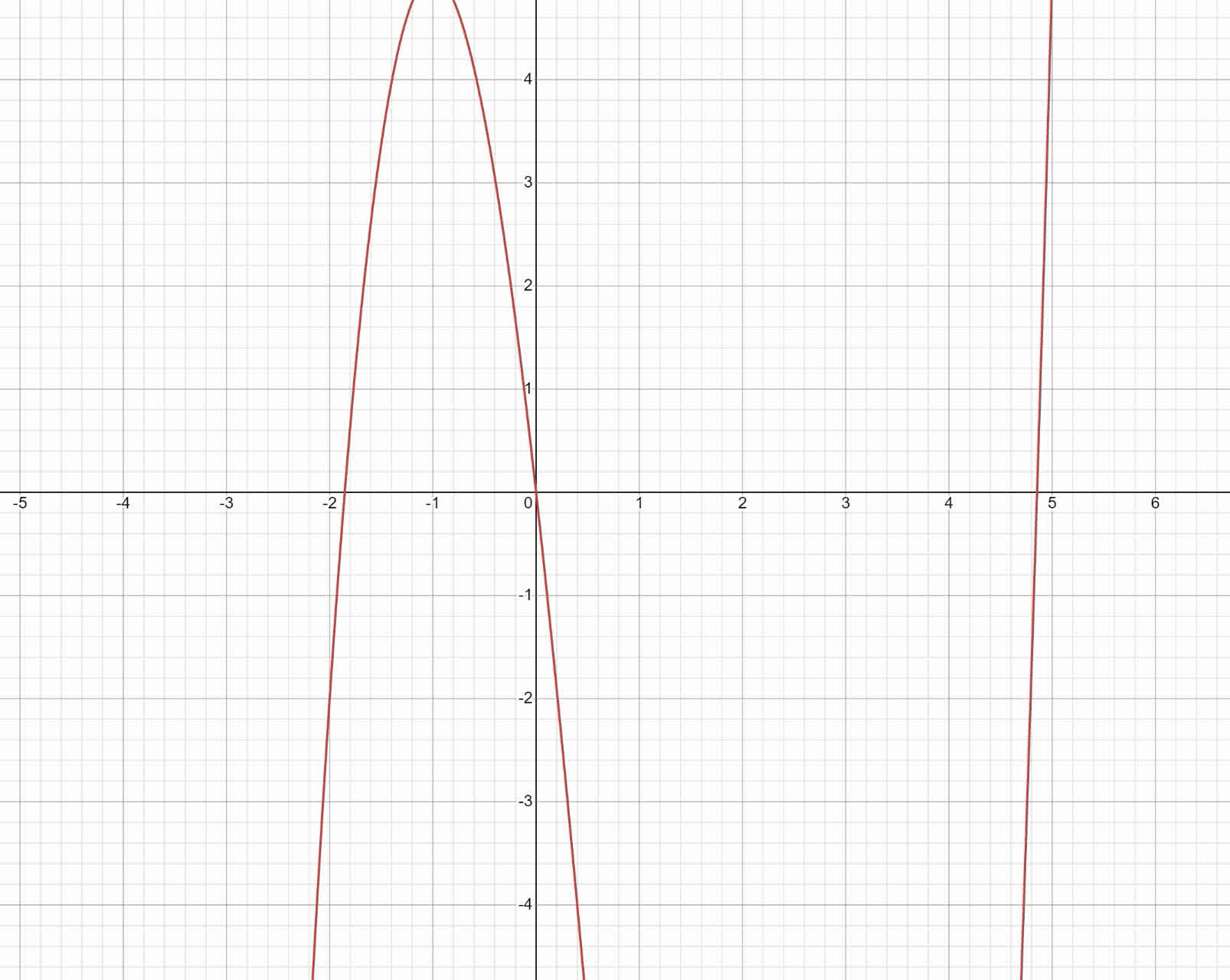Nann

2021-08-18

In each item, do the following analytically: (a) find the relative extrema of f. (b) determine the values of x at which the relative extrema occur. (c) determine the intervals on which f is increasing. (d) determine the intervals on which f is decreasing. $f\left(x\right)={x}^{3}-3{x}^{2}-9x$StrycharzT

(a) Find the domain of the funtion Finde the derivative ${f}^{\prime }\left(x\right)=3{x}^{2}-6x-9$ Find the domain of derivative Substitute f'(x)=0 $0=3{x}^{2}-6x-9$ Solve equation $x=-1$
$x=3$
Determine th intervals $⟨-\mathrm{\infty },-1⟩,⟨-1,3⟩$
$⟨-1,3⟩,⟨3,+\mathrm{\infty }⟩$ Choose the points ${x}_{1}=-2$
${x}_{2}=0$
${x}_{3}=0$
${x}_{4}=0$ Calculate the values of the derivatives ${f}^{\prime }\left(-2\right)=15$
${f}^{\prime }\left(0\right)=-9$
${f}^{\prime }\left(0\right)=-9$
${f}^{\prime }\left(4\right)=15$ The relative maximum at x=-1 The relative minimum is at x=3
Calculate the value $f\left(-1\right)=5$
f(3)=-27 The relative maximum of the function is 5 at x=-1 The relative minimum of the function is -27 at x=3 (b) To find x-intercept, substitute f(x)=0 $0={x}^{3}-3{x}^{2}-9x$ Swap the sides ${x}^{3}-3{x}^{2}-9x=0$ Factor the expression $x×\left({x}^{2}-3x-9\right)=0$ Separate onto possible cases $x=0$
${x}^{2}-3x-9=0$ Solve the equation $x=0$
$x=\frac{3+3\sqrt{5}}{2}$
$x=\frac{3-3\sqrt{5}}{2}$ There is 3 solutions (c) and (d) Let's plot the polynomial to determine the intervals of increasing and decreasing. Increase by: $\left(-\mathrm{\infty },-1\right),\left(3,\mathrm{\infty }\right)$ Decreases by: $\left(-1,3\right)$Do you have a similar question?